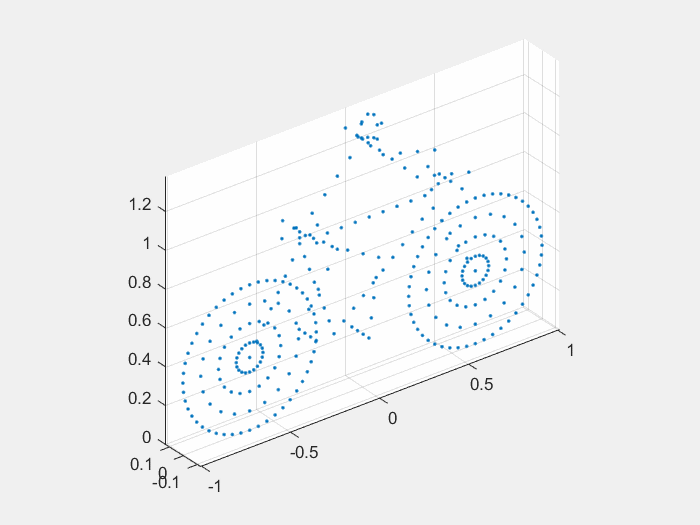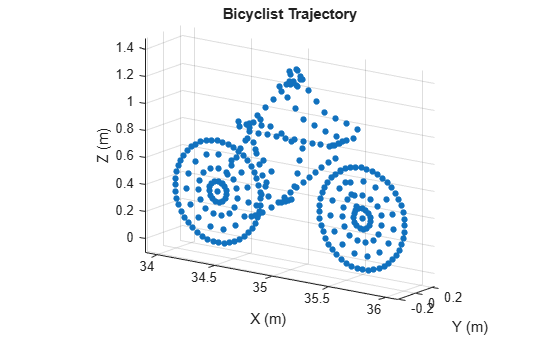# backscatterBicyclist

Since R2021a

## Description

The `backscatterBicyclist` object simulates backscattered radar signals reflected from a moving bicyclist. The bicyclist consists of both the bicycle and its rider. The object models the motion of the bicyclist and computes the sum of all reflected signals from multiple discrete scatterers on the bicyclist. The model ignores internal occlusions within the bicyclist. The reflected signals are based on a multi-scatterer model developed from a 77 GHz radar system.

Scatterers are located on five major bicyclist components:

• Bicycle frame and rider

• Bicycle pedals

• Upper and lower legs of the rider

• Front wheel

• Back wheel

Excluding the wheels, there are 114 scatterers on the bicyclist. The wheels contain scatterers on the rim and spokes. The number of scatterers on the wheels depends on the number of spokes per wheel. The number of spokes is specified using the `NumWheelSpokes` property.

You can obtain the current bicyclist position and velocity by calling the `move` object function. Calling this function also updates the position and velocity for the next time epoch. To obtain the reflected signal, call the `reflect` object function. You can plot the instantaneous position of the bicyclist using the `plot` object function.

## Creation

### Syntax

```bicyclist = backscatterBicyclist bicyclist = backscatterBicyclist(Name,Value,...) ```

### Description

`bicyclist = backscatterBicyclist` creates a `backscatterBicyclist` object, `bicyclist`, having default property values.

`bicyclist = backscatterBicyclist(Name,Value,...)` creates a `backscatterBicyclist` object, `bicyclist`, with each specified property `Name` set to the specified `Value`. You can specify additional name-value pair arguments in any order as (`Name1`,`Value1`,...,`NameN`,`ValueN`). Any unspecified properties take default values. For example,

```bicyclist = backscatterBicyclist( ... 'NumWheelSpokes',18,'Speed',10.0, ... 'InitialPosition',[0;0;0],'InitialHeading',90, ... 'GearTransmissionRatio',5.5);```
models a bicycle with 18 spokes on each wheel that is moving along the positive y-axis at 10 meters per second. The gear transmission ratio of 5.5 indicates that there are 5.5 wheel rotations for each pedal rotation. The bicyclist is heading along the y-axis.

This figure illustrates a bicyclist starting to turn left.## Properties

expand all

Number of spokes per wheel of the bicycle, specified as a positive integer from 3 to 50, inclusive.

Data Types: `double`

Ratio of wheel rotations to pedal rotations, specified as a positive scalar. The gear ratio must be in the range from 0.5 through 6. Units are dimensionless.

Data Types: `double`

Carrier frequency of the narrowband incident signals, specified as a positive scalar. Units are in Hz.

Example: `900e6`

Data Types: `double`

Initial position of the bicyclist, specified as a 3-by-1 real-valued vector in the form of [x;y;z] in global coordinates. Units are in meters. The initial position corresponds to the location of the origin of the bicycle coordinates. The origin is at the center of mass of the scatterers of the default bicyclist configuration projected onto the ground.

Data Types: `double`

Initial heading of bicyclist, specified as a scalar. Heading is measured in the xy-plane from the x-axis towards the y-axis. Heading is with respect to global coordinates. Units are in degrees.

Data Types: `double`

Speed of bicyclist, specified as a nonnegative scalar. The motion model limits the speed to a maximum of 60 m/s (216 kph). Speed is defined with respect to global coordinates. Units are in meters per second.

Data Types: `double`

Set bicycle coasting state, specified as `false` or `true`. If set to `true`, the bicyclist is not pedaling, but the wheels are still rotating (freewheeling). If set to `false`, the bicyclist is pedaling, and the `GearTransmissionRatio` determines the wheel rotations to pedal rotations.

Data Types: `logical`

Signal propagation speed, specified as a positive scalar. Units are in meters per second. The default propagation speed is the value returned by `physconst('LightSpeed')`. See `physconst` for more information.

Example: `3e8`

Data Types: `double`

Radar cross-section azimuth angles, specified as a 1-by-P or P-by-1 real-valued vector. This property defines the azimuth coordinates of each column of the radar cross-section matrix specified by the `RCSPattern` property. P must be greater than two. Angle units are in degrees.

Example: `[-45:0.1:45]`

Data Types: `double`

Radar cross-section elevation angles, specified as a 1-by-Q or Q-by-1 real-valued vector. This property defines the elevation coordinates of each row of the radar cross-section matrix specified by the `RCSPattern` property. Q must be greater than two. Angle units are in degrees.

Example: `[-30:0.1:30]`

Data Types: `double`

Radar cross-section (RCS) pattern, specified as a Q-by-P real-valued matrix or a 1-by-P real-valued vector. Matrix rows represent constant elevation, and columns represent constant azimuth. Q is the length of the vector defined by the `ElevationAngles` property. P is the length of the vector defined by the `AzimuthAngles` property. Units are in square meters.

You can also specify the pattern as a 1-by-P real-valued vector of azimuth angles for a single elevation.

The default value of this property is a 1-by-361 matrix containing values derived from 77 GHz radar measurements of a bicyclist. The default values of `AzimuthAngles` and `ElevationAngles` correspond to the default RCS matrix.

Example: `[1,.5;.5,1]`

Data Types: `double`

## Object Functions

expand all

 `getNumScatterers` Number of scatterers on bicyclist `move` Position, velocity, and orientation of moving bicyclist `plot` Display locations of scatterers on bicyclist `reflect` Reflected signal from moving bicyclist
 `step` Run System object algorithm `release` Release resources and allow changes to System object property values and input characteristics `reset` Reset internal states of System object

## Examples

collapse all

Compute the backscattered radar signal from a bicyclist moving along the x-axis at 5 m/s away from a radar. Assume that the radar is located at the origin. The radar transmits an LFM signal at 24 GHz with a 300 MHz bandwidth. A signal is reflected at the moment the bicyclist starts to move and then one second later.

Initialize Bicyclist, Waveform, and Propagation Channel Objects

Initialize the `backscatterBicyclist`, `phased.LinearFMWaveform`, and `phased.FreeSpace` objects. Assume a 300 MHz sampling frequency. The initial position of the bicyclist lies on the x-axis 30 meters from the radar.

```bw = 300e6; fs = bw; fc = 24e9; radarpos = [0;0;0]; bpos = [30;0;0]; bicyclist = backscatterBicyclist( ... 'OperatingFrequency',fc,'NumWheelSpokes',15, ... 'InitialPosition',bpos,'Speed',5.0, ... 'InitialHeading',0.0); lfmwav = phased.LinearFMWaveform( ... 'SampleRate',fs, ... 'SweepBandwidth',bw); sig = lfmwav(); chan = phased.FreeSpace( ... 'OperatingFrequency',fc, ... 'SampleRate',fs, ... 'TwoWayPropagation',true);```

Plot Initial Bicyclist Position

Using the `move` object function, obtain the initial scatterer positions, velocities and the orientation of the bicyclist. Plot the initial position of the bicyclist. The `dt` argument of the `move` object function determines that the next call to `move` returns the bicyclist state of motion `dt` seconds later.

```dt = 1.0; [bpos,bvel,bax] = move(bicyclist,dt,0); plot(bicyclist)```Obtain First Reflected Signal

Propagate the signal to all scatterers and obtain the cumulative reflected return signal.

```N = getNumScatterers(bicyclist); sigtrns = chan(repmat(sig,1,N),radarpos,bpos,[0;0;0],bvel); [rngs,ang] = rangeangle(radarpos,bpos,bax); y0 = reflect(bicyclist,sigtrns,ang);```

Plot Bicyclist Position After Position Update

After the bicyclist has moved, obtain the scatterer positions and velocities and then move the bicycle along its trajectory for another second.

```[bpos,bvel,bax] = move(bicyclist,dt,0); plot(bicyclist)```Obtain Second Reflected Signal

Propagate the signal to all scatterers at their new positions and obtain the cumulative reflected return signal.

```sigtrns = chan(repmat(sig,1,N),radarpos,bpos,[0;0;0],bvel); [~,ang] = rangeangle(radarpos,bpos,bax); y1 = reflect(bicyclist,sigtrns,ang);```

Match Filter Reflected Signals

Match filter the reflected signals and plot them together.

```mfsig = getMatchedFilter(lfmwav); nsamp = length(mfsig); mf = phased.MatchedFilter('Coefficients',mfsig); ymf = mf([y0 y1]); fdelay = (nsamp-1)/fs; t = (0:size(ymf,1)-1)/fs - fdelay; c = physconst('LightSpeed'); plot(c*t/2,mag2db(abs(ymf))) ylim([-200 -50]) xlabel('Range (m)') ylabel('Magnitude (dB)') ax = axis; axis([0,100,ax(3),ax(4)]) grid legend('First pulse','Second pulse')```Compute the difference in range between the maxima of the two pulses.

```[maxy,idx] = max(abs(ymf)); dpeaks = t(1,idx(2)) - t(1,idx(1)); drng = c*dpeaks/2```
```drng = 4.9965 ```

The range difference is 5 m, as expected given the bicyclist speed.

Display a spectrogram showing the micro-Doppler effect on radar signals reflected from the scatterers on a moving bicyclist target. A stationary radar transmits 1000 pulses of an FMCW radar wave with a bandwidth of 250 MHz and of 1 $\mu \mathrm{sec}\text{\hspace{0.17em}}$duration. The radar operates at 24 GHz. The bicyclist starts 5 m from the radar and moves away at 4 m/s.

Set up the waveform, channel, transmitter, receiver, and platform System objects.

```bw = 250e6; fs = 2*bw; fc = 24e9; c = physconst('Lightspeed'); tm = 1e-6; wav = phased.FMCWWaveform('SampleRate',fs,'SweepTime',tm, ... 'SweepBandwidth',bw); chan = phased.FreeSpace('PropagationSpeed',c,'OperatingFrequency',fc, ... 'TwoWayPropagation',true,'SampleRate',fs); radarplt = phased.Platform('InitialPosition',[0;0;0], ... 'OrientationAxesOutputPort',true); trx = phased.Transmitter('PeakPower',1,'Gain',25); rcvx = phased.ReceiverPreamp('Gain',25,'NoiseFigure',10);```

Create a `bicyclist` object moving at 4 meters/second.

```bicyclistSpeed = 4; bicyclist = backscatterBicyclist('InitialPosition',[5;0;0],'Speed',bicyclistSpeed, ... 'PropagationSpeed',c,'OperatingFrequency',fc,'InitialHeading',0.0); lambda = c/fc; fmax = 2*bicyclist.GearTransmissionRatio*bicyclistSpeed/lambda; tsamp = 1/(2*fmax);```

Loop over 1000 pulses. Find the angle of incidence of the radar. Propagate the wave to each scatterer, and then reflect the wave from the scatterers back to the radar.

```npulse = 1000; xr = complex(zeros(round(fs*tm),npulse)); for m = 1:npulse [posr,velr,axr] = radarplt(tsamp); [post,velt,axt] = move(bicyclist,tsamp,0); [~,angrt] = rangeangle(posr,post,axt); x = trx(wav()); xt = chan(repmat(x,1,size(post,2)),posr,post,velr,velt); xr(:,m) = rcvx(reflect(bicyclist,xt,angrt)); end```

Process the arriving signals. First, dechirp the signal and then pass the signal into a Kaiser-windowed short-time Fourier transform.

```xd = conj(dechirp(xr,x)); M = 128; beta = 6; w = kaiser(M,beta); R = floor(1.7*(M-1)/(beta+1)); noverlap = M - R; [S,F,T] = stft(sum(xd),1/tsamp,'Window',w,'FFTLength',M*2, ... 'OverlapLength',noverlap); maxval = max(10*log10(abs(S))); pcolor(T,-F*lambda/2,10*log10(abs(S))-maxval); shading flat; colorbar xlabel('Time (sec)') ylabel('Speed (m/s)')```Create a custom RCS pattern to use with the `backscatterBicyclist` object.

The RCS pattern is computed from cosines raised to the fourth power. Plot the pattern.

```az = [-180:180]; el = [-90:90]; caz = cosd(az').^4; cel = cosd(el).^4; rcs = (caz*cel)'; imagesc(az,el,rcs) xlabel('Azimuth (deg)') ylabel('Elevation (deg)') colorbar``````bicyclist = backscatterBicyclist( ... 'NumWheelSpokes',18,'Speed',10.0, ... 'InitialPosition',[0;0;0],'InitialHeading',90, ... 'GearTransmissionRatio',5.5,'AzimuthAngles',az, ... 'ElevationAngles',el,'RCSPattern',rcs);```

## Algorithms

expand all

 Stolz, M. et al. "Multi-Target Reflection Point Model of Cyclists for Automotive Radar." 2017 European Radar Conference (EURAD), Nuremberg, 2017, pp. 94–97.

 Chen, V., D. Tahmoush, and W. J. Miceli. Radar Micro-Doppler Signatures: Processing and Applications. The Institution of Engineering and Technology: London, 2014.

 Belgiovane, D., and C. C. Chen. "Bicycles and Human Rider Backscattering at 77 GHz for Automotive Radar." 2016 10th European Conference on Antennas and Propagation (EuCAP), Davos, 2016, pp. 1–5.

 Victor Chen, The Micro-Doppler Effect in Radar. Norwood, MA: Artech House, 2011.